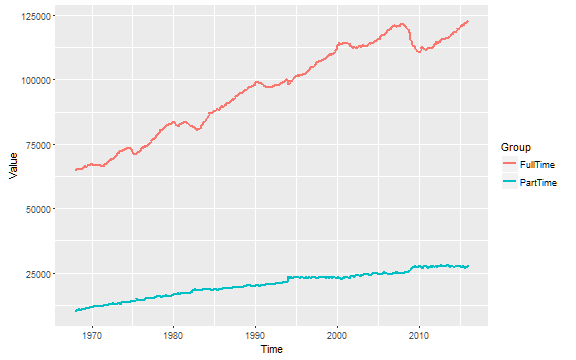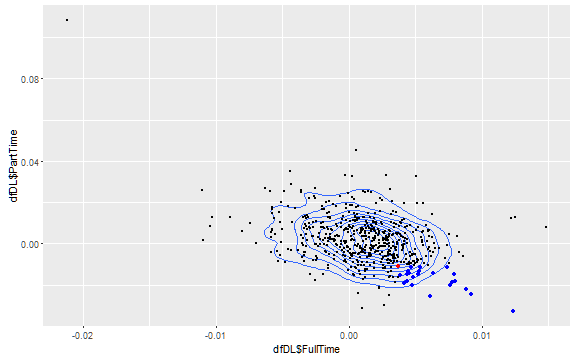# Re: Data Paranoia – maybe justified this time?

Menzie Chinn responded to a post on Zero Hedge in which someone claimed that the september spread in the gain of full-time jobs and the loss in part-time jobs would be unusually high. Menzie responded that this would be well within the probability given the history of these time series. Thankfully he gave links to the data on the FRED database. As I was wasting the time anyway, I thought I would take a look at the data.

##  "LNS12600000" "LNS12500000"So, we have a set of 549 montly data from January 1968 to September 2013. As in Menzie’s piece, i take the first difference of the logs to get monthly rates of change.

dfDL = as.data.frame(apply(df, 2, function(x)diff(log(x))))
names(dfDL) = c("FullTime", "PartTime")
summary(dfDL)

##     FullTime             PartTime
##  Min.   :-0.0211697   Min.   :-0.032792
##  1st Qu.:-0.0006873   1st Qu.:-0.005594
##  Median : 0.0013157   Median : 0.001420
##  Mean   : 0.0011179   Mean   : 0.001760
##  3rd Qu.: 0.0032261   3rd Qu.: 0.007903
##  Max.   : 0.0148232   Max.   : 0.108558


We define the september 2013 values as cutfff-points, and get the data points with an even more extreme spread between full-time and part-time.

# In case someone would try to use the code at a later time.
sep13 = which(index(LNS12500000)=="2013-09-01")-1
cutOffs = dfDL[sep13,]
lowerRight = dfDL[which(dfDL$FullTime > cutOffs$FullTime &
dfDL$PartTime< cutOffs$PartTime),]


Looking at the scatter-plot, we could expect a negative correlation between the two variablesAnd this is confirmed, the correlation is -0.3918705. So, on first glance it would not seem to be unlikely that there should be a large increase of full-time jobs and a large decrease in part-time jobs. In fact, it would be the expected result that, given a high value for one series, we have high value for the other series with the opposite sign.

Identifying the empirical likelyhood of the event, we see that the red dot is September 2013, blue ones are larger spreads – 5 points, which gives us a frequency for this event of 1.0928962%. Next step is to see, if the results is statistically more unlikely than what we have observed.

We assume a bivariate normal distribution with observed mean values and covariance matrix:

mu = colMeans(dfDL)
sigma = cov(dfDL)
dfSum = data.frame(Mean = mu, StDev = apply(dfDL,2,sd))
dfSum

##                 Mean       StDev
## FullTime 0.001117893 0.003495736
## PartTime 0.001760472 0.011738748


As we can see, the mean rate of the part-time series is about 80% higher than the full-time series. It also has a much larger standard deviation.

To calculate the probability of the joint event that the full-time-rate in a given month is geq 0.3702089% and that the part-time-rate in a given month is leq -1.1011538% we put the numbers into R:

probEvent = pmvnorm(upper =unlist(c(Inf,cutOffs)),lower =unlist(c(cutOffs,-Inf)),mean = mu, sigma = sigma)


And the result is 6.2379699% – or about once very 1.3359047 years, which is in the same ballpark-range as the observed frequency of 1.0928962% or once every 7.625 years.

We contrast it with the outlier on the other side of the distribution, which looks like a rebasement in Januar 1994, as we have a decrease of -2078.83973288225 in the number of full-time jobs and an increase of 2576.9469075262 in the number of part-time-jobs.

we get the following results:

whichPT = which.max(dfDL\$PartTime)
cutOffs2 = dfDL[whichPT,]
cutOffs2

##               FullTime  PartTime
## 1994-01-01 -0.02116966 0.1085579

probEvent2 = pmvnorm(lower =unlist(c(-Inf,cutOffs2)),upper =unlist(c(cutOffs2,Inf)),mean = mu, sigma = sigma)


And the result is 6.1032592 × 10-21% – which is a “not in the lifetime of the universe”-kind of likelihood.

So, the answer to the leading question – is the paranoia justified this time – is no. This month’s development is not statistically unlikely, especially in contrast to a genuine man-made event.